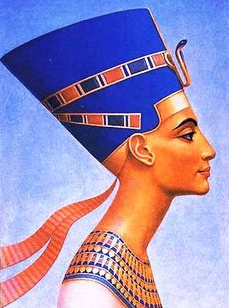Sullivan Library at WDMS: Grade 6 Research Guides

Ancient Civilizations

Grade 6: Ancient Civilizations^^^^^^^^^^^^^^^^^^^^^^^^^^^^^^^^^^^^^^^^^^^^^^^^^^^^^^^^^^^^^^^^^^^^^^^^^^^^^^^^^^^^^^^^^^^^^^^^^^^^^^^^^^^^^^^^^^^^^^^^^^^^^^^^^^^^^^^^^^^^^^^^^^^^^^^Website Evaluation - Just CART it! A successful researcher always evaluates information, especially from the Internet.  Diamond students use the CART method.

Real or Fake?

What do you think? Would you trust the information on these websites?

Website Evaluation ActivityCART  Lesson

Students will work in pairs to
evaluate 2 different websites
using the CART method.

Be prepared to explain why one website is a better resource than the other.

Each pair will need:
1 CART checklist & 1 ChromebookGROUP A - Queen Nefertiti
website #1
website #2GROUP B - Ziggurat